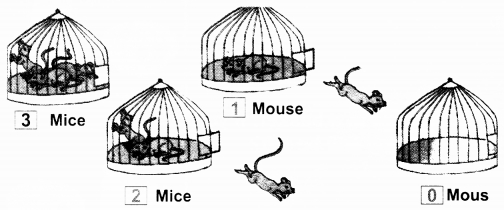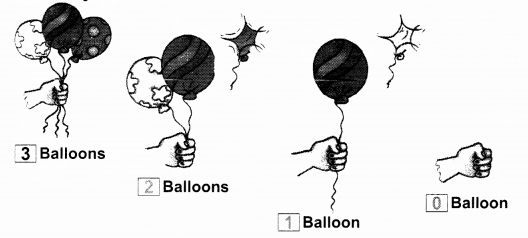NCERT Solutions for Class 1 Maths Chapter 2 Numbers from One to Nine includes all the important topics with detailed explanation that aims to help students to understand the concepts better. Students who are preparing for their Class 1 exams must go through NCERT Solutions for Class 1 Maths Chapter 2 Numbers from One to Nine. Going through the solutions provided on this page will help you to know how to approach and solve the problems.

Students can also find NCERT intext, exercises and back of chapter questions. Also working on Class 1 Mathematics Chapter 2 Numbers from One to Nine NCERT Solutions will be most helpful to the students to solve their Homeworks and Assignments on time. Students can also download NCERT Solutions for Class 1 Maths Chapter 2 Numbers from One to Nine PDF to access them even in offline mode.

Board: CBSE
Textbook: NCERT
Class: Class 1
Subject: Maths (Math Magic)
Chapter Name: Numbers from One to Nine
Number of Questions – 29

## NCERT Solutions for Class 1 Maths Chapter 2 Numbers from One to Nine

NCERT Solutions for Class 1 Maths Chapter 1 Shapes and Space are been solved by top teachers of LearnCBSE.in. All the solutions given in this page are solved based on CBSE Syllabus & NCERT guidelines. So before getting into the details of CBSE NCERT Solutions for Class 1 Maths Magic Chapter 1 Shapes and Space let’s have an overview of list of topics and sub topics under this chapter.

• As many as
• Counting
• More or Less
• Count and Match
• Numbers from One to Nine
• Make groups of
• Match the numbers with the pictures.
• Colour the collection which has more objects.
• Make the collection equal.
• Join in order.
• Write the missing number.
• Count and write the number of birds on the branch.

NCERT Textbook Page 24
More or Less

Question 1.
Tick (✓) more.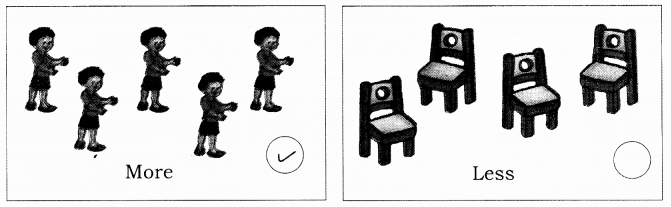Question 2.
Tick (✓) less.Question 3.
Tick (✓) less.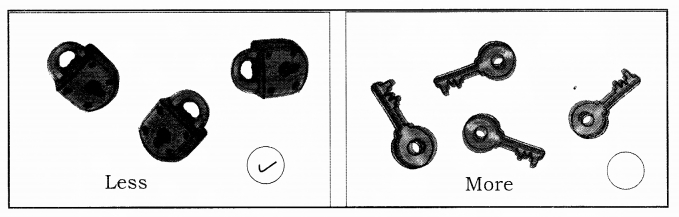Question 4.
Tick (✓) more.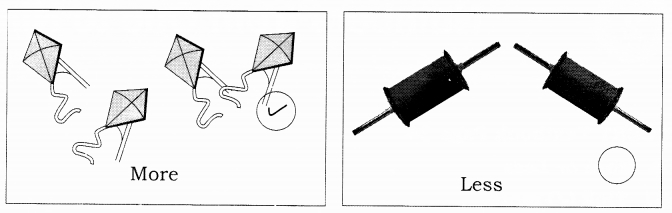NCERT Textbook Page 25
Count and Match

Question 1.
Count and match the following :Question 2.
Count and match the following :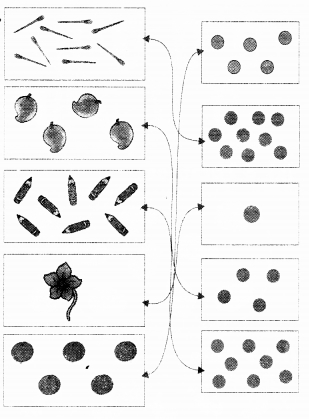Question 3.
Count and match the following :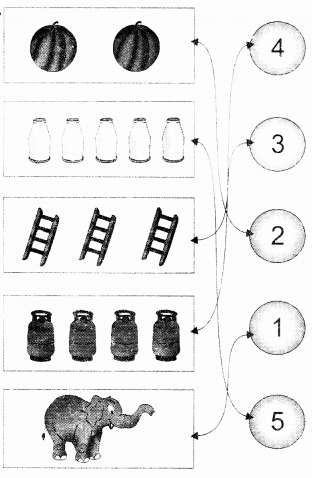Question 4.
Count and match the following :Question 5.
Match the numbers with the pictures :Question 6.
Make groups of the following :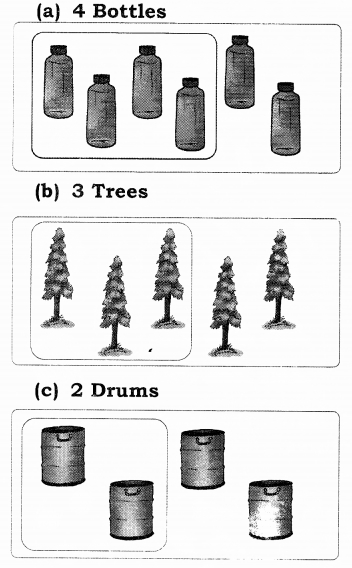Question 7.
Colour the following :

(a) Colour 5 balloons.(b) Colour 4 flowers(c) Colour 2 trees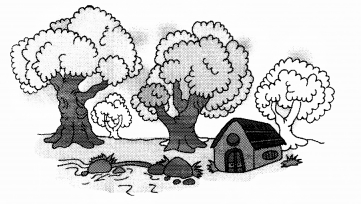(d) Colour 3 boats(e) Colour 6 birds(f) Colour 9 mangoes(g) Colour 1 elephant(h) Colour 7 bricksNCERT Textbook page 43

Question 1.
Name these things and write their number in the circle :Question 2.
Colour the collection which has more objects.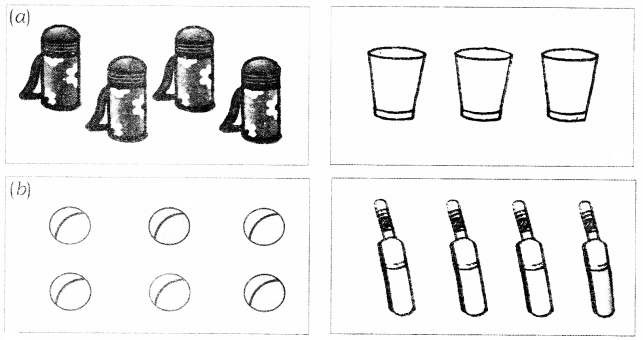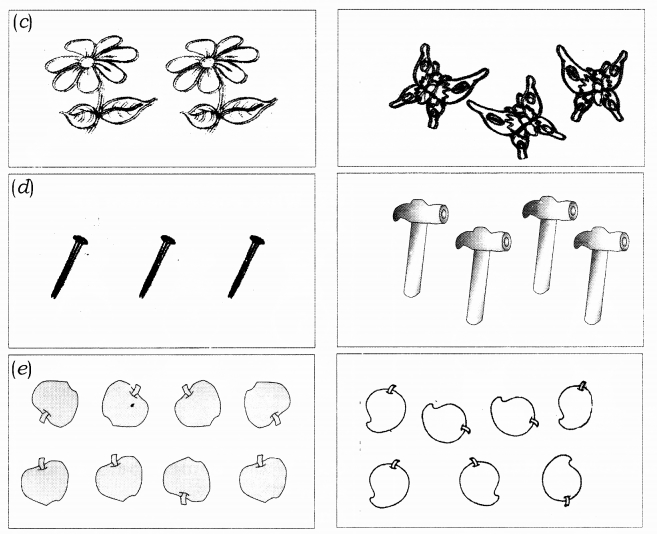Question 3.
Make the collection equal.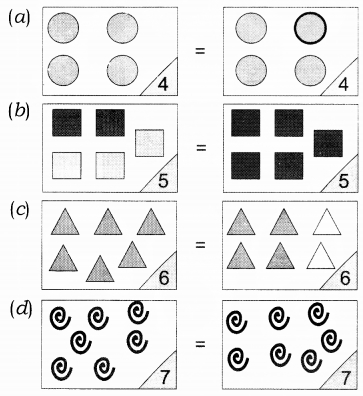Question 4.
Join in order :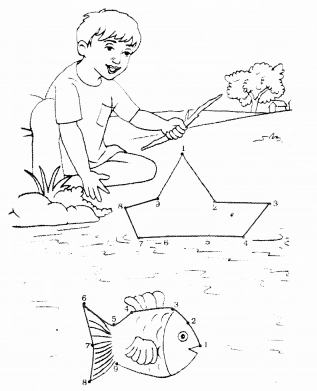Question 5.
Write the missing number.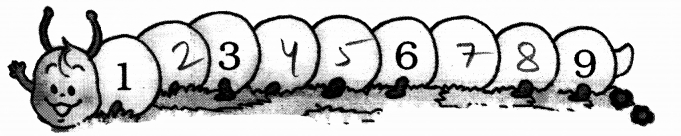Question 6.
What comes before and after 5?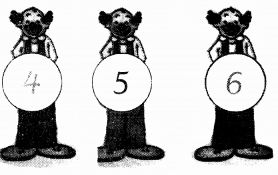Question 7.
What comes before 6?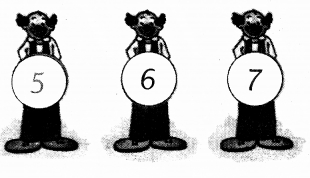Question 8.
What comes after 1?Question 9.
What comes before and after 4?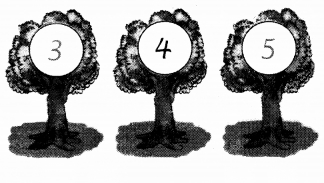Question 10.
What are the next numbers?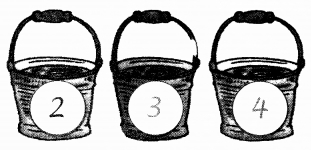Question 11.
What are the next numbers?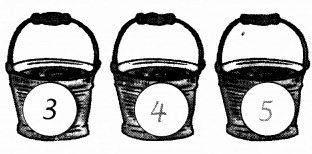NCERT Textbook Page 48
Zero

Question 1.
Five juicy mangoes are kept in a basket. If you pick and eat one, how many will be left?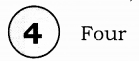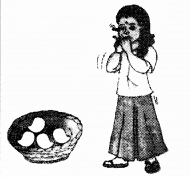Question 2.
Four juicy mangoes are kept in a basket. If you pick and eat one, how many will be left?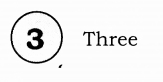Question 3.
Three juicy mangoes are kept in a basket. If you pick and eat one, how many will be left?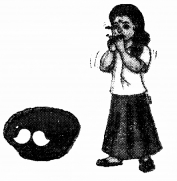Question 4.
Two juicy mangoes are kept in a basket. If you pick and eat one, how many will be left?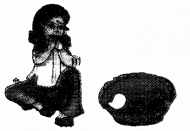Question 5.
One juicy mango is kept in a basket. If you pick and eat one, how many will be left?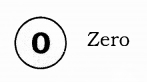Question 1.
Count and write the number of birds on the branch.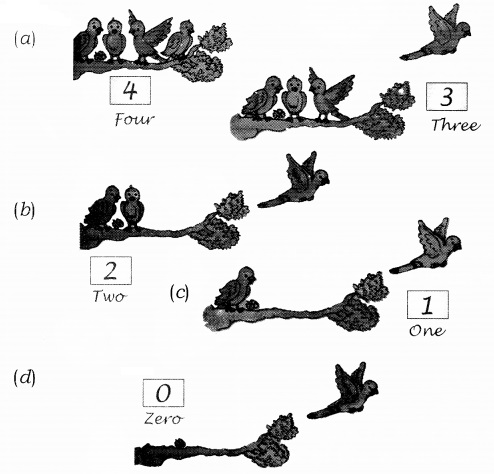Question 2.
How many in the cage?